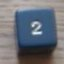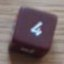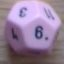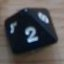How to generate a perfect bell curve?

## How to generate a perfect bell curve?

Return to topic list

 Mon Mar 24 13:20:47 2014   by   Daniel Hi, I want to generate a perfect bell curve of an hypothetical perfect dice roll, to compare with my dice machanic...its possible with troll? Tue Mar 25 09:20:48 2014   by   Torben What is normally called a bell curve is continuous distribution galled the normal distribution which is the limit of many discrete distributions (see http://en.wikipedia.org/wiki/Normal_distribution#The_central_limit_theorem).  Troll handles only discrete distributions, but you can get close to a normal distribution by adding many dice (as shown in the Wikipedia article).For example, sum 100z1 will give you a pretty good approximation centered around 50. Tue Mar 25 14:05:50 2014   by   Daniel My dice mechanic have 1 to 16 of range, and a virtual 8.5 of average...It makes a discrete curve, but have any ways to check mathematically how close my curve is of a perfect normal distribution using troll? Sorry if I ask an off-topic subject... Wed Mar 26 08:58:50 2014   by   Torben Normal distributions have infinite range, and there are infinitely many that are centered around 8.5.  The difference is the spread.  So if you want to test how close your distribution is to a normal distribution, you should test it against a normal distribution with the same spread as yours.  If you tell me your spread, I can try to cook up an approximate normal distribution with the same spread. Wed Mar 26 15:36:02 2014   by   Daniel Now Im testing two variations of this mechanic, first with d8's and second with d12's. You need to change the "x" to choose about these variations.[code]x:= 12;if d2 = 1thenmax 3d xelsemin 3d x + x[/code]With d8 the average is 8.5, and spread 2.54644359843.With d12 the average is 12.5, and spread 3.80575830766Thanks for this big help! Wed Mar 26 17:20:50 2014   by   Torben For the d12 variant, a reasonably close approximation is `sum 59z1 - 17` which has an average of 12.5 and a spread of 3.84.For the d8 variant, `sum 25z1 - 4` has an average of 8.5 and a spread of 2.5. Thu Mar 27 23:45:33 2014   by   Daniel Thanks!

Return to topic list

New message:
 Topic: Posted by:

Type the values of the dice shown below:Return to topic list